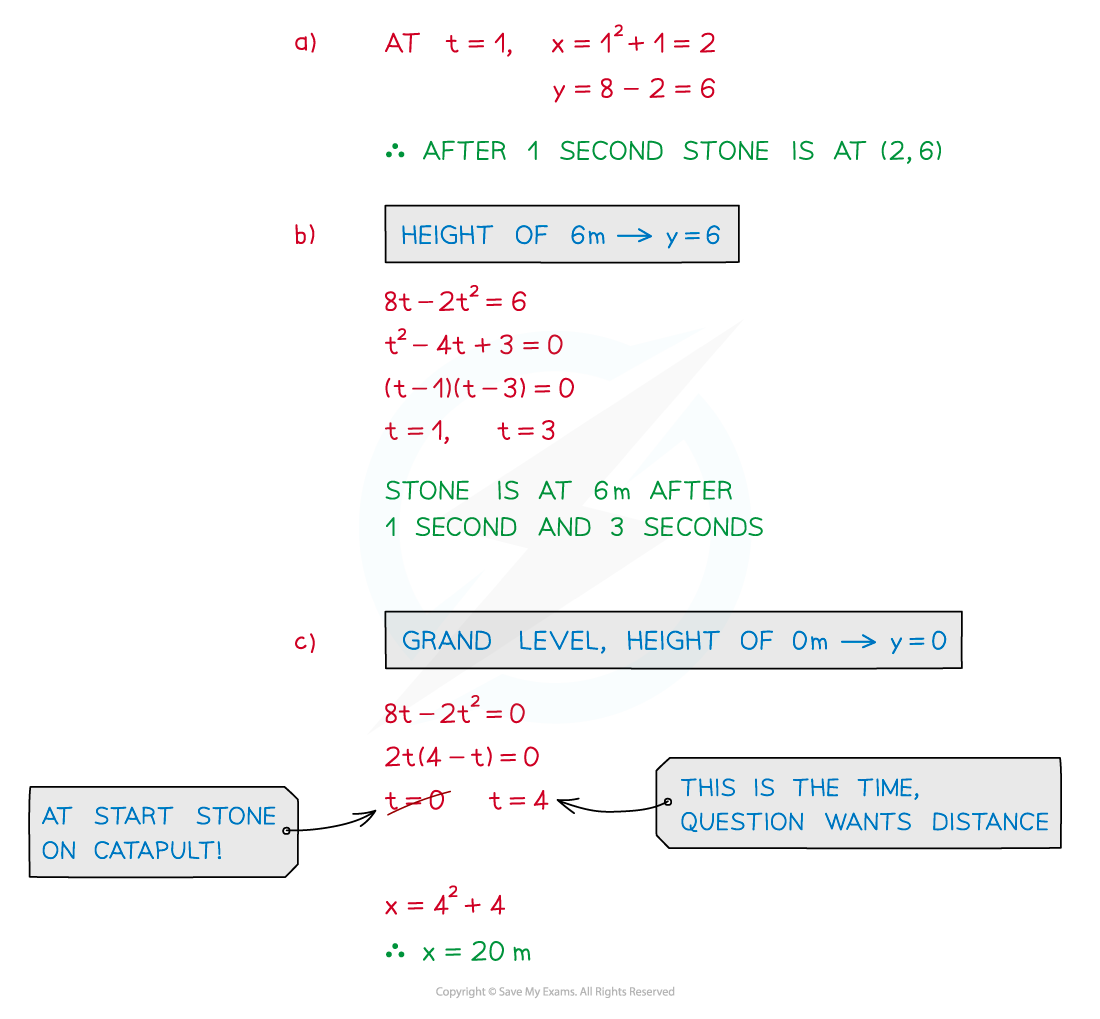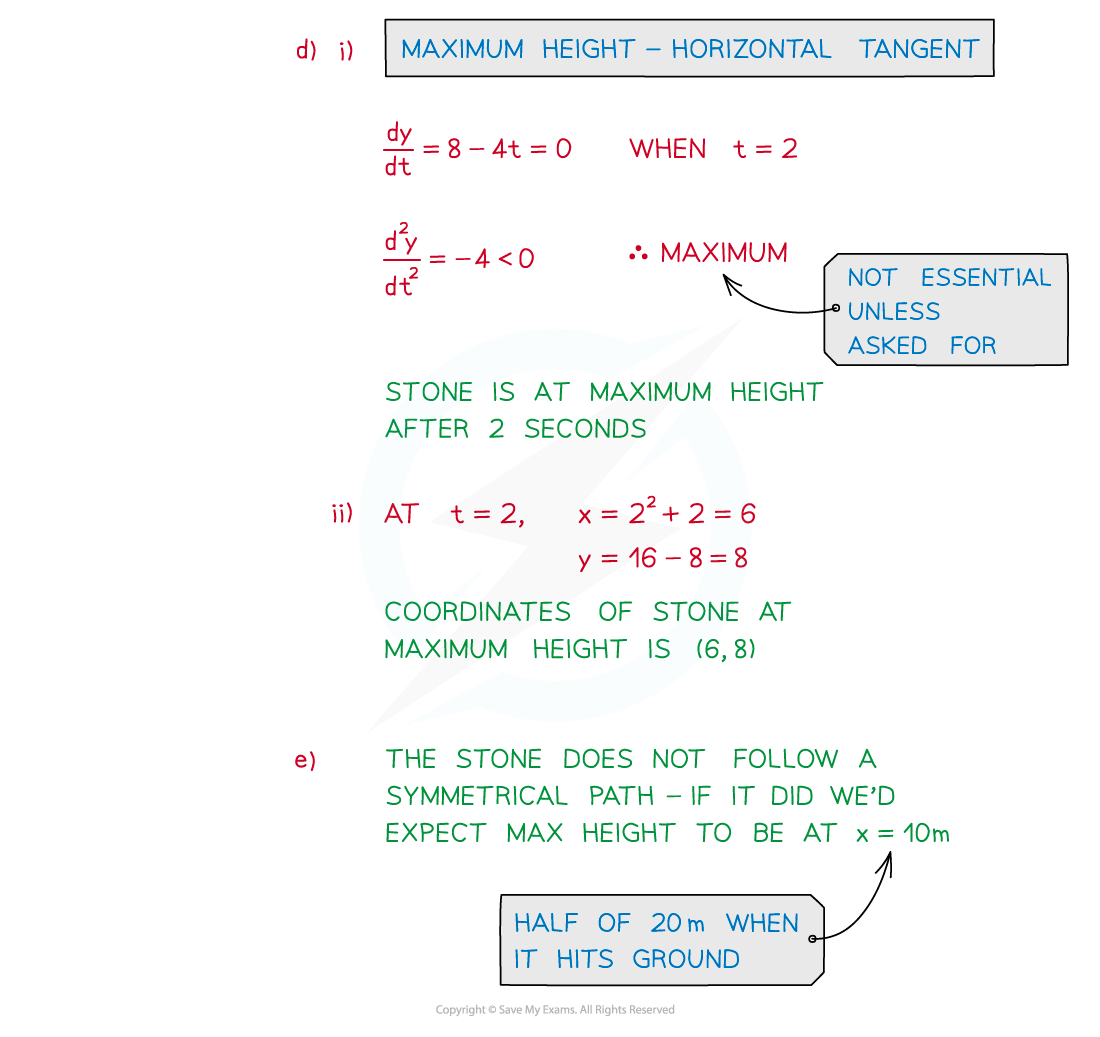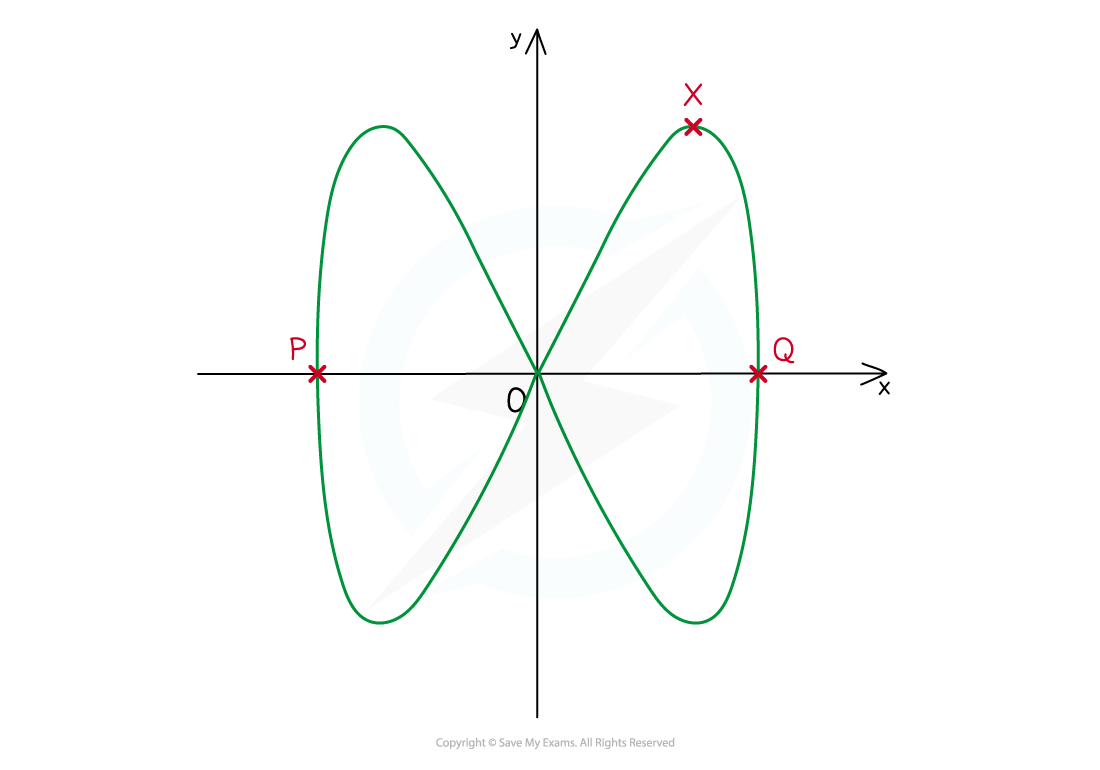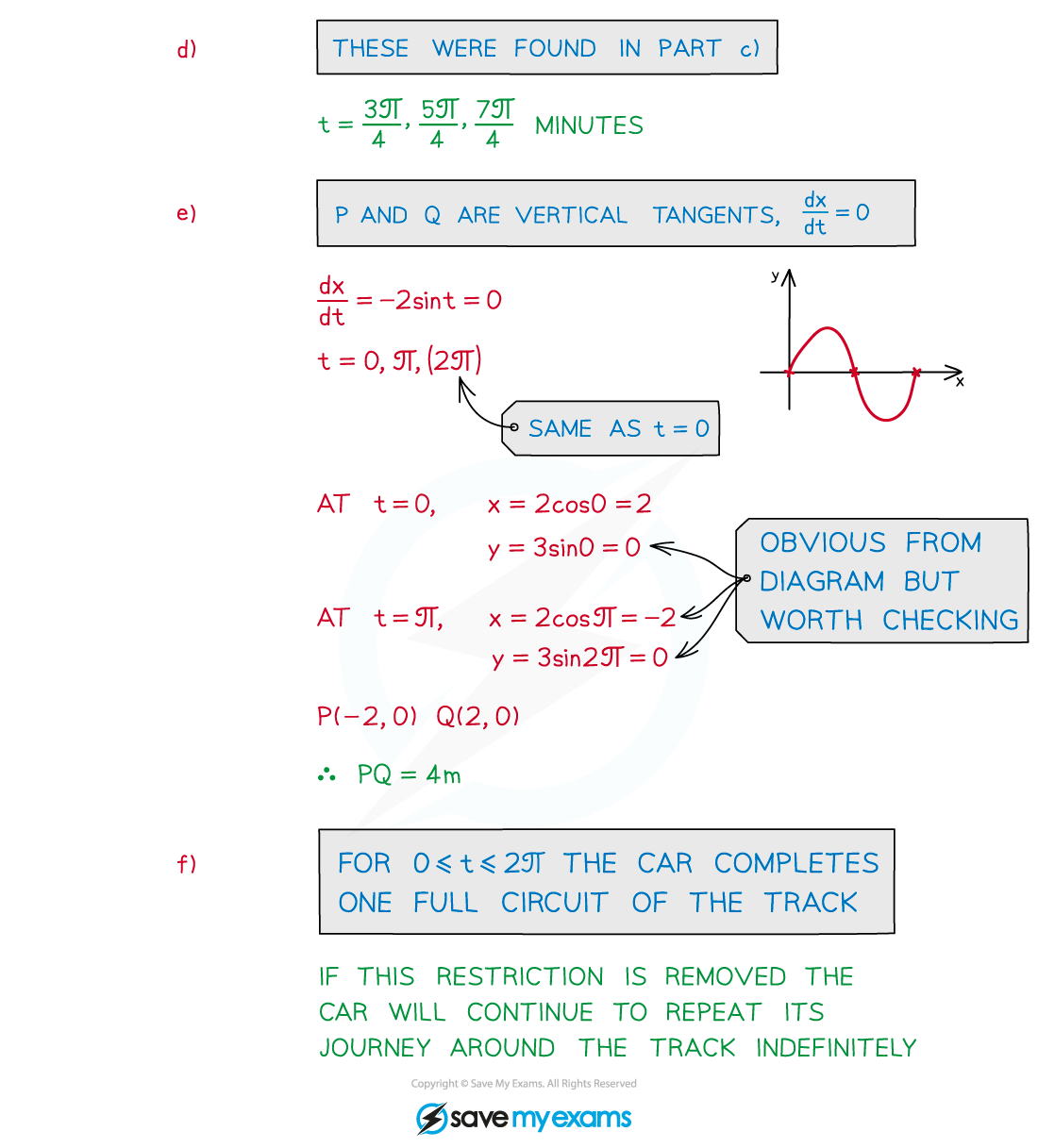# AQA A Level Maths: Pure复习笔记9.2.3 Modelling with Parametric Equations

### Modelling with Parametric Equations

#### What can be modelled with parametric equations?

• A common use for parametric equations is motion in two dimensions
• x = f(t) – horizontal position, dependent on time
• y = f(t) – vertical position, dependent on time
• This is explored in vectors and projectiles#### Worked Example